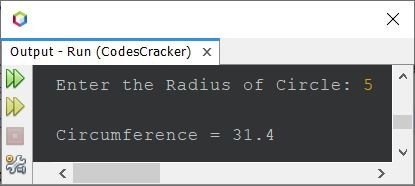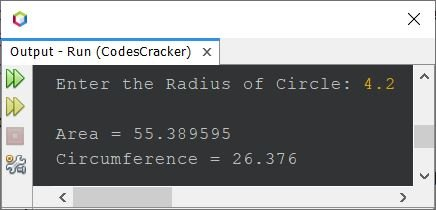# Java Program to Calculate Area and Circumference of a Circle

This article covers a program in Java that find and prints area and circumference of a circle. Both area and circumference will get calculated based on the radius provided at run-time of the program.

Note - The area of a circle is calculated using the formula 3.14*r*r. Where r is the radius value of circle.

Note - The circumference of a circle is calculated using the formula 2*3.14*r. Where r is the radius value of circle.

Note - In above formulae, 3.14 is the value of Pi or π

## Find Area of Circle in Java

The question is, write a Java program to calculate area of circle. The answer to this question is the program given below:

```import java.util.Scanner;

public class CodesCracker
{
public static void main(String[] args)
{
float r, area;
Scanner s = new Scanner(System.in);

System.out.print("Enter the Radius of Circle: ");
r = s.nextFloat();

area = (float)(3.14*r*r);
System.out.println("\nArea = " +area);
}
}```

The snapshot given below shows the sample run of above Java program with user input 5 as radius of that circle, whose area we want to find and print using the program:In the following statement, of above program:

`area = (float)(3.14*r*r);`

the float() is used to convert whatever the value comes out from 3.14*r*r, into floating-point, to avoid Incompatible Error, i.e., possible loosy conversion from double to float. That is, because the type of area variable is declared as float, therefore we need to convert the value comes from 3.14*r*r into float

## Find Circumference of Circle in Java

The question is, write a Java program to calculate circumference of a circle. The program given below is its answer:

```import java.util.Scanner;

public class CodesCracker
{
public static void main(String[] args)
{
float r, circum;
Scanner s = new Scanner(System.in);

System.out.print("Enter the Radius of Circle: ");
r = s.nextFloat();

circum = (float)(2*3.14*r);
System.out.println("\nCircumference = " +circum);
}
}```

Here is its sample run with same user input as of previous program. That is, 5 as radius of circle:## Calculate Area and Circumference of Circle in Java

This is basically the combined version of previous two programs. That is, I've combined both the program, so that in a single program, we can see the area and circumference both at one execution of the program.

```import java.util.Scanner;

public class CodesCracker
{
public static void main(String[] args)
{
float r, area, circum;
Scanner s = new Scanner(System.in);

System.out.print("Enter the Radius of Circle: ");
r = s.nextFloat();

area = (float)(3.14*r*r);
circum = (float)(2*3.14*r);
System.out.println("\nArea = " +area);
System.out.println("Circumference = " +circum);
}
}```

The sample run of above program, with user input 4.2, is:#### Same Program in Other Languages

Java Online Test

« Previous Program Next Program »